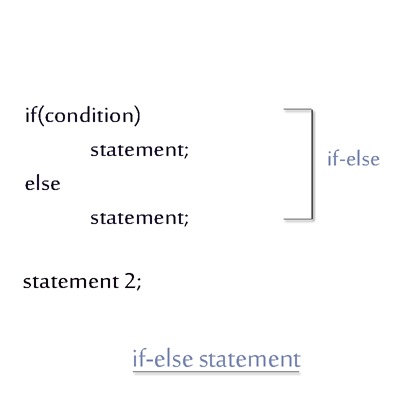< Prev
Next >

# C++ If Else Statement

In the if-else statement of C++ language, the condition in the parenthesis of if is evaluated for a boolean value - true or false.

• If this condition is true then the statement or a block of statements connected to the if statement is executed.

• If this condition is false then the statement or a block of statements connected to the else is executed.• ## if else example

• `````` /* C++ if else example */

#include<iostream>

using namespace std;
int main()
{
if(10<50)
cout<<"10 is less than 50";
else
cout<<"10 is greater than 50";

return 0;
}``````

## Output -

``10 is less than 50``

In the program above, we have compared two int values using an if-else statement to find which integer value is greater.

• ## Another if else example

``````/* C++ if else example */

#include<iostream>

using namespace std;
int main()
{
//Declaring multiple char variables.
char a = 'a', b = 'b';

//An if-else statement comparing values in char variables
if(a<b)
cout<<"a is less than b";
else
cout<<"b is greater than a";

return 0;
}``````

## Output -

``a is less than b``

In the latter program, we have compared values in char variables using an if-else statement, internally their ASCII values have been compared to find out which character has a greater ASCII value.

< Prev
Next >
< If Statement
Else-If Statement>